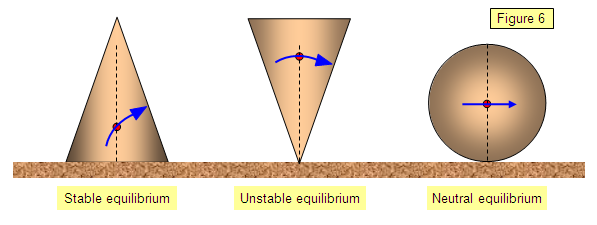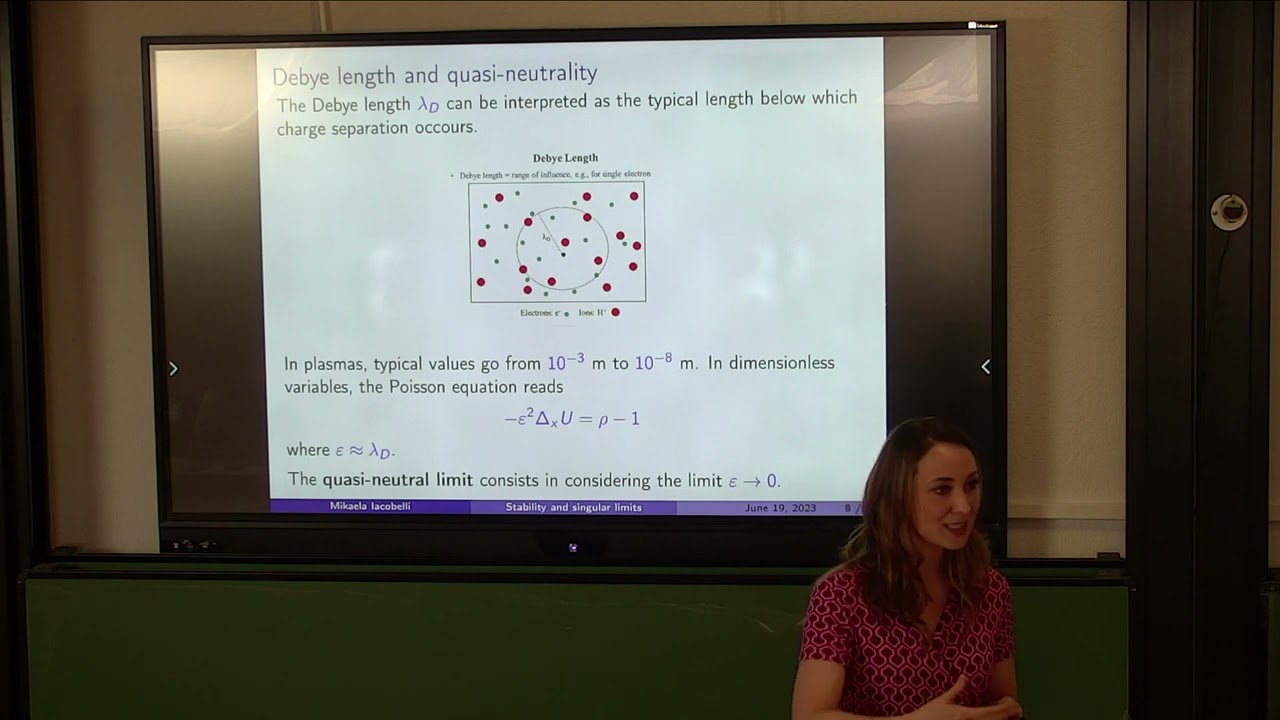Connect with us

# Concept of Neutral Stability: A Comprehensive ExplorationPublished

onThe idea of stability is essential to the fields of science, mathematics, and engineering. It serves as the foundation for comprehending the behavior of many different kinds of systems, including natural processes and physical constructions. Although the concept of “stability” is frequently linked to both stability and instability, there is an interesting intermediate state known as “neutral stability.” This difficult idea adds a fresh perspective to our comprehension of balance and equilibrium in a variety of systems.

We shall explore neutral stability in depth in this article, looking at its concept, mathematical representation, and application to many academic subjects. Readers ought to fully understand this fascinating idea and its ramifications by the conclusion.

## What Neutral Stability Means

It is crucial to first grasp what stability implies in the context of systems, equations, and phenomena in order to fully understand the idea of neutral stability.The ability of a system or process to regain equilibrium following a disturbance is referred to as stability in the broadest meaning. When exposed to outside forces, stable systems often stay near their equilibrium points, while unstable systems show significant deviations and are unable to reach equilibrium again.

Between these two extremes, neutral stability serves as a transitional stage where a system neither goes back to its equilibrium nor strays from it, but rather teeters on the edge of instability, preserving an unstable balance.

### Computational Illustration

An essential component of comprehending neutral stability is knowing how it is represented mathematically. We use linear systems theory, where linearization is a widely used method for assessing the stability of dynamic systems, to articulate it.Imagine a system that is defined by a system of differential equations, usually expressed as x’ = f(x), where f is the vector field that defines the dynamics of the system, x is the state vector, and x’ is its derivative. By approximating the vector field f with a linear function, usually using the Jacobian matrix J, linearization makes these equations simpler.

x’ = Jx, where J is the Jacobian matrix assessed at the equilibrium point x*, is the linearized system’s formula.

We now look at the eigenvalues of the Jacobian matrix J to ascertain the stability of this linearized system. These eigenvalues give insights into the behavior of the system by representing its characteristic roots. The fundamental requirement for neutral stability is that the real component of at least one of J’s eigenvalues must equal zero.

An equilibrium condition devoid of exponential fluctuations is reached when the real component of an eigenvalue is zero, indicating that the corresponding mode in the system neither grows nor decays over time.

In conclusion, when the real component of at least one eigenvalue of a system equals zero, the system is said to be neutrally stable. This term in mathematics provides a basis for evaluating neutral stability in different fields.

## Neutral Stability in Engineering and Physics

### Mechanical Systems:

The study of equilibrium points and vibrational modes is one area in which neutral stability is applied in mechanical engineering. For illustration, think about a pendulum. It is in a state of neutral stability when it is at rest, hanging downward vertically. The pendulum will swing with any slight disturbance, but it won’t swing higher or lower forever; instead, it will oscillate back and forth while holding its neutral equilibrium position.### Control Theory:

It is essential to comprehend the behavior of systems under control in control systems engineering. When a control system is intended to keep a specific setpoint, reaching absolute stability may not be the goal. Without exponential growth or instability, neutral stability enables the controlled system to hold a desired position.

### Electrical Circuits:

Resonant circuits in electrical engineering exhibit neutral stability. For example, when the values of the capacitance (C) and inductance (L) are selected so that the driving frequency and resonant frequency exactly match, the system can be neutrally stable in a basic LC circuit. The voltage and current oscillate in this case, but they do not increase or decrease exponentially.

### Fluid Dynamics:

Neutral stability is understood in terms of flow instability and boundary layers in fluid mechanics. The flow maintains neutral stability when the Reynolds number reaches a crucial point. Any perturbation added to the flow will continue steadily without increasing or decreasing over time.## In Mathematical Stability, Neutrality

### Differential Equations:

Neutral stability is a useful idea in the field of differential equations while researching linear time-invariant systems. It sheds light on how solutions behave close to equilibrium points, particularly when certain modes behave neutrally.

### Stability Analysis:

Since neutral stability denotes the boundary between stability and instability, it is important to take it into account while using stability analysis methodologies. For example, a system is said to be stable in the context of Lyapunov stability if it meets specific requirements, such as Lyapunov functions. On the other hand, neutral stability occurs when the Lyapunov function reaches a constant value along a trajectory, indicating that there is neither attraction nor repulsion between solutions in the system.## Conclusion:

Neutral stability is a concept that broadens our understanding of balance and equilibrium in a variety of systems. In this condition, a system neither returns to its equilibrium nor deviates from it; rather, it exists as the boundary between stability and instability. Comprehending is essential in domains spanning from environmental sciences and mathematics to engineering and physics.

The idea of neutral stability will definitely be important as we investigate and study complex systems in order to forecast and manage their behavior. It challenges us to consider the subtle nuances of equilibrium, offering insights into how systems maintain while exhibiting dynamic changes. Neutral stability is a reminder that the world around us is often more intricate and finely balanced than it may initially appear, and it is a testament to the beauty of science and mathematics in unraveling such complexity.

Advertisement